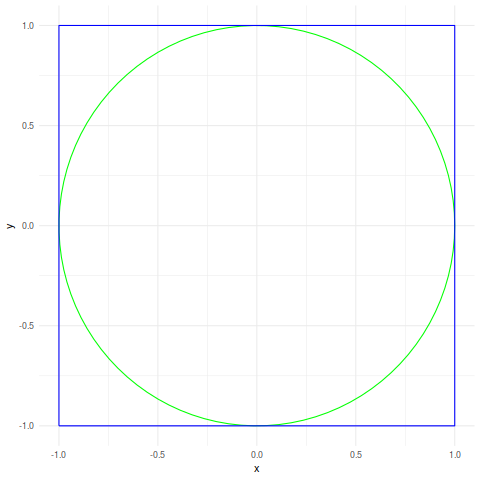# The Curse of Dimensionality (Again)

Posted on August 29, 2019

This note is partly a test of my org-babel/hakyll setup and partly and exploration of a new (to me) form of the curse of dimensionality.

What is the curse of dimensionality? Imagine a circle of radius 1 and the surrounding square.

``````library(ggplot2)

circleFun <- function(center = c(0,0),radius = 1, npoints = 100){
tt <- seq(0,2*pi,length.out = npoints)
xx <- center + radius * cos(tt)
yy <- center + radius * sin(tt)
return(data.frame(x = xx, y = yy))
}

circle <- circleFun()
square <- data.frame(x=c(-1,1,1,-1,-1),y=c(-1,-1,1,1,-1))

ggplot(circle,aes(x=x,y=y)) +
geom_path(color="green") +
geom_path(data=square,color="blue") +
theme_minimal()``````The circle takes up pi/4 ~= 79% of the square.

What happens in three dimensions? The volume of the cube with sides of length 2 is 8. The volume of a sphere with radius 1 is (4/3)pi so which is 52% of the volume of the containing cube.

This pattern continues as the number of dimensions increases:

Dimensions Fraction of volume
1 100%
2 79%
3 52%
4 31%
5 16%
6 8%

This is the curse of dimensionality - in higher dimensions almost all the volume of a hypercube is in the corners.

For a normally distributed data, roughly 95% of the observations will be within two standard deviations of the mean (actually 1.96 standard deviations, but who’s counting?).

This is only true for one dimensional observations. In higher dimensions, the curse of dimensionality strikes again and the proportion is a lot lower.

``````  makeSamples <- function(n, max.dim) {
## Allocate an empty matrix
output <- matrix(, nrow=n, ncol=max.dim)
## Fill each column with normally distributed data
for (i in 1:max.dim) {
samples <- rnorm(n,0,1)
output[,i] <- samples
}
return(output)
}

samples <- makeSamples(1000,10)

result <- data.frame(dimensions=1:10,
proportion_within_2_sd=NA_real_
)

result\$proportion_within_2_sd <- sum(abs(samples[,1]) < 2)/1000

for (i in 2:10) {
distance <- apply(samples[,1:i],1,FUN = function(x) {sqrt(sum(x*x))})
proportion <- sum(distance < 2)/1000 # 1000 samples
result\$proportion_within_2_sd[i] <- proportion
}

library(knitr)
kable(result,"markdown",
col.names = c("Dimensions",
"Proportion within 2 s.d.")
)``````
Dimensions Proportion within 2 s.d.
1 0.953
2 0.869
3 0.741
4 0.601
5 0.467
6 0.324
7 0.221
8 0.147
9 0.103
10 0.063

For multivariate linear regression (where there is more than one response variable) a smaller and smaller proportion of the residuals will be “small” in some sense.

[O]ur intuitions, which come from a three-dimensional world, often do not apply in high-dimensional ones. In high dimensions, most of the mass of a multivariate Gaussian distribution is not near the mean, but in an increasingly distant “shell” around it; and most of the volume of a high-dimensional orange is in the skin, not the pulp. If a constant number of examples is distributed uniformly in a high-dimensional hypercube, beyond some dimensionality most examples are closer to a face of the hypercube than to their nearest neighbor. And if we approximate a hypersphere by inscribing it in a hypercube, in high dimensions almost all the volume of the hypercube is outside the hypersphere. This is bad news for machine learning, where shapes of one type are often approximated by shapes of another.

A Few Useful Things to Know About Machine Learning by Pedro Domingos

I most recently came across this problem after presenting a kmeans cluster analysis to a client. I was asked “how close are the points in the cluster to the centre?” which is easy enough to answer. But then the follow up question was “and is this good?”

Each column of the data was scaled to have a mean of zero and standard deviation of one so it intuitively felt like there should be a global answer to this; e.g. if 95% of the observations are within 2 s.d. of the cluster centre then this is good. But the curse of dimensionality means that even with quite a low number of dimensions the proportion within a fixed distance of the cluster centre will be small.

As far as I know, there isn’t one. Using non-Euclidean distance metrics can help but only up to a certain point. Here is the same table from above but using a fractional norm:

``````  result <- data.frame(dimensions=1:10,
proportion_within_2_sd=NA_real_
)

result\$proportion_within_2_sd <- sum(abs(samples[,1]) < 2)/1000

for (i in 2:10) {
distance <- apply(samples[,1:i],1,FUN = function(x) { sum(abs(x)^6)^(1/6)})
proportion <- sum(distance < 2)/1000 # 1000 samples
result\$proportion_within_2_sd[i] <- proportion
}

library(knitr)
kable(result,"markdown",
col.names = c("Dimensions",
"Proportion within 2 s.d.")
)``````
Dimensions Proportion within 2 s.d.
1 0.953
2 0.902
3 0.850
4 0.806
5 0.753
6 0.701
7 0.651
8 0.603
9 0.553
10 0.501

But this only delays the problem - by the time we get to 20 dimensions things will be just as bad as they were before (or worse).

The other alternative is to reduce the dimensionality somehow. PCA can work well for this